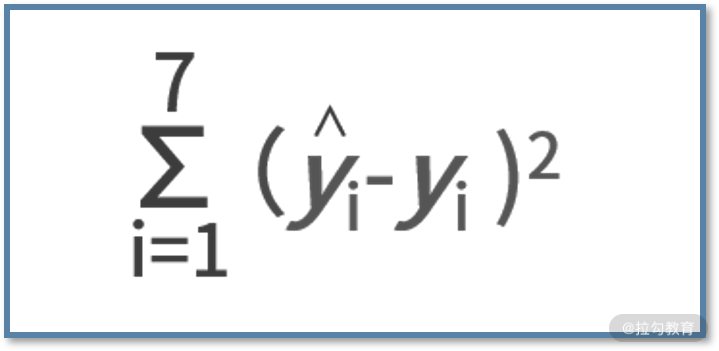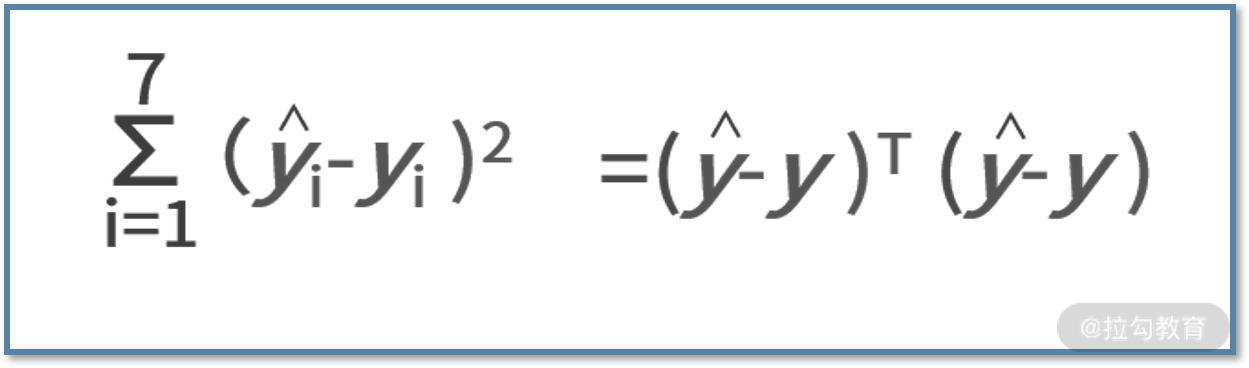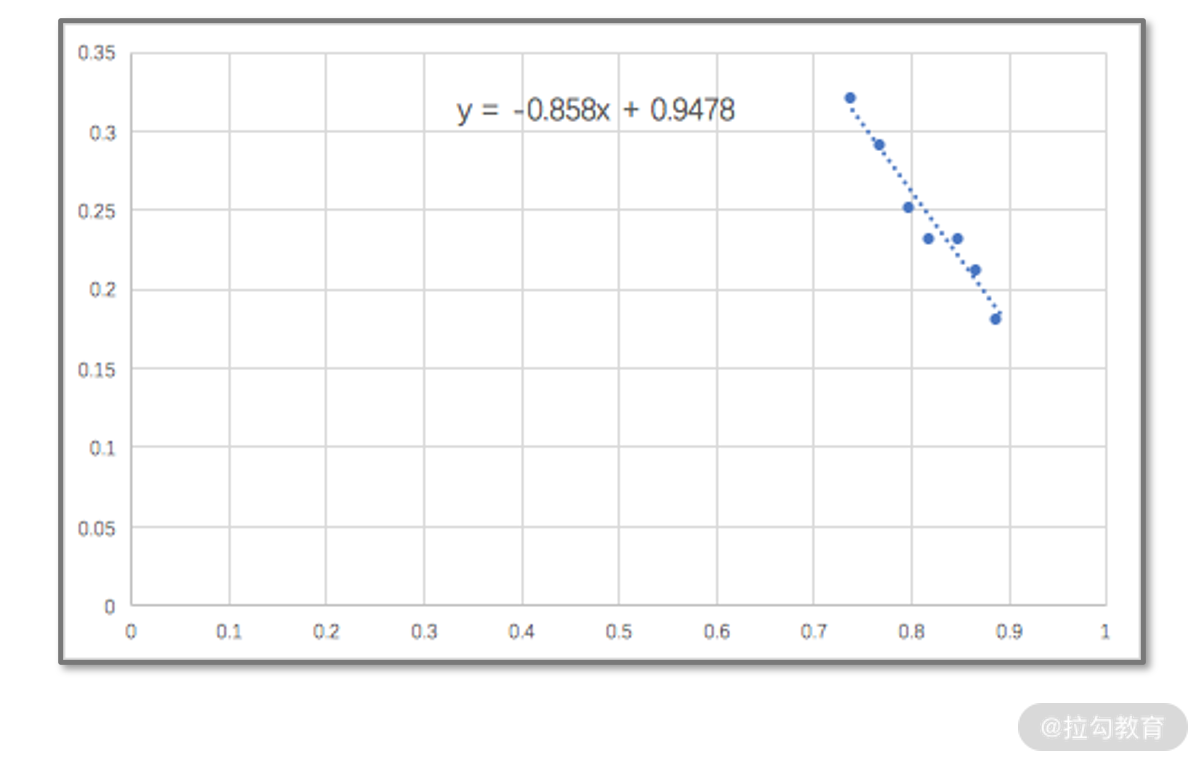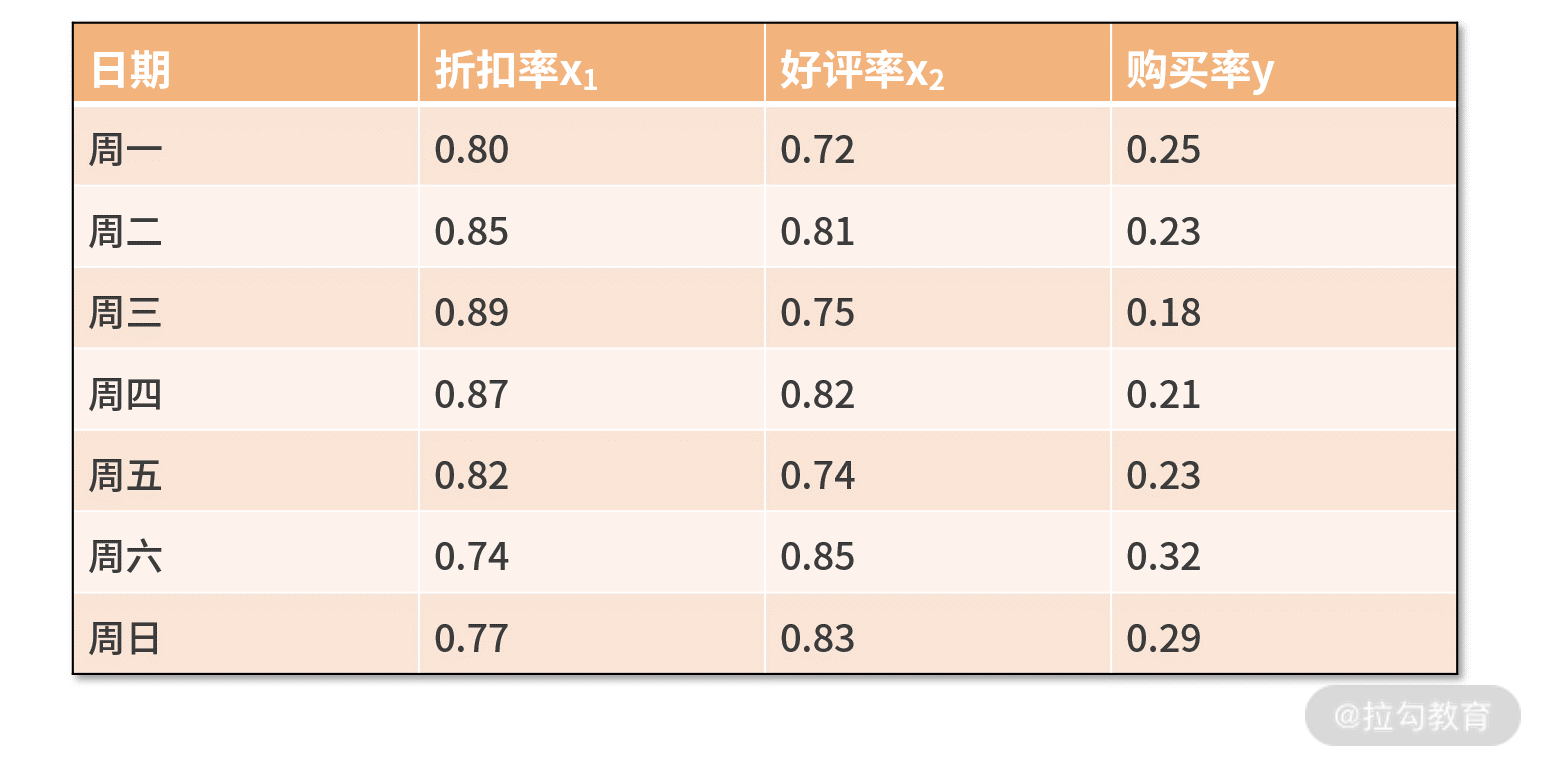07 线性回归：如何在离散点中寻找数据规律？

y =kTx+b。

线性回归的形式化定义线性回归的求解方法线性回归编程实战

import numpy as np

def main():

x = np.array([[0.80,1],[0.85,1],[0.89,1],[0.87,1],[0.82,1],[0.74,1],[0.77,1]])

yhat = np.array([[0.25],[0.23],[0.18],[0.21],[0.23],[0.32],[0.29]])

xtx = np.dot(x.T,x)

xtx_1 = np.linalg.inv(xtx)

w = xtx_1.dot(x.T).dot(yhat)

print 'k: ' + str(w)

print 'b: ' + str(w)

if __name__ == '__main__':

main()

• 第 4 行，导入数据得到矩阵 X。为了还能求解出 b，我们需要对每个 xi 补充一个“1”；
• 第 5 行，导入数据得到真实值向量 ŷ。接下来，按照公式进行求解就可以了；
• 第 6 行，计算了 XTX 的结果；
• 第 7 行，对其求逆，得到了（XTX）-1；
• 第 8 行，再来对 XT 和 ŷ 计算矩阵乘法，得到最终的 w思维发散

• 普通程序员会写代码，一流的程序员懂数学。

• 既然 Excel 这么强大，我能否不学数学，而用 Excel 来打天下呢？import numpy as np

def main():

x = np.array([[0.80,0.72,1],[0.85,0.81,1],[0.89,0.75,1],[0.87,0.82,1],[0.82,0.74,1],[0.74,0.85,1],[0.77,0.83,1]])

yhat = np.array([[0.25],[0.23],[0.18],[0.21],[0.23],[0.32],[0.29]])

xtx = np.dot(x.T,x)

xtx_1 = np.linalg.inv(xtx)

w = xtx_1.dot(x.T).dot(yhat)

print 'k1: ' + str(w)

print 'k2: ' + str(w)

print 'b: ' + str(w)

if __name__ == '__main__':

main()

小结

• 自己去造一些数据，分别利用 Excel 和自己写的代码，亲自试一下线性回归的拟合；
• 如果我们不采用求导法，而采用梯度下降法，试着写一下代码吧。